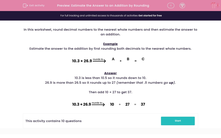### Comprehensive & curriculum aligned

In this worksheet, students round decimal numbers to the nearest whole numbers in order to estimate the answer to an addition.Key stage:  KS 2

Curriculum topic:   Maths and Numerical Reasoning

Curriculum subtopic:   Decimals

Difficulty level:#### Worksheet Overview

In this worksheet, round decimal numbers to the nearest whole numbers and then estimate the answer to an addition.

Example

Estimate the answer to the addition by first rounding both decimals to the nearest whole numbers.

10.3 26.9A
+
 B
=
 C

10.3 is less than 10.5 so it rounds down to 10.

26.9 is more than 26.5 so it rounds up to 27 (remember that .5 numbers go up).

Then add 10 + 27 to get 37.

10.3 26.910
+
 27
=
 37

### What is EdPlace?

We're your National Curriculum aligned online education content provider helping each child succeed in English, maths and science from year 1 to GCSE. With an EdPlace account you’ll be able to track and measure progress, helping each child achieve their best. We build confidence and attainment by personalising each child’s learning at a level that suits them.

Get started